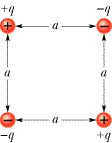# Problem: How much work in joules is required to set up the four-charge configuration of the figure if q = 4.10 pC, a = 74.0 cm, and the particles are initially infinitely far apart and at rest?

###### FREE Expert Solution

The work required is given by the change in potential energy for the four charge configuration.

That is,

$\overline{){\mathbf{W}}{\mathbf{=}}{\mathbf{∆}}{\mathbf{U}}}$, where W is work and U is potential energy.

The electrostatic potential energy:

$\overline{){\mathbf{U}}{\mathbf{=}}\frac{\mathbf{k}{\mathbf{q}}_{\mathbf{1}}{\mathbf{q}}_{\mathbf{2}}}{\mathbf{r}}}$, where k is Coulomb's constant and r is the distance between two charges q1 and q2.

91% (62 ratings)###### Problem Details

How much work in joules is required to set up the four-charge configuration of the figure if q = 4.10 pC, a = 74.0 cm, and the particles are initially infinitely far apart and at rest?